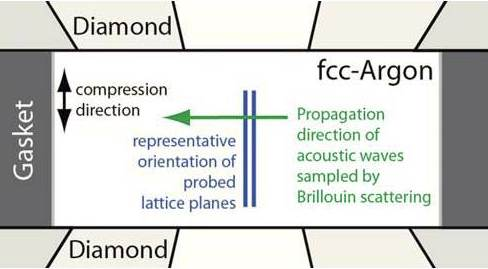Select Page

We performed simultaneous Brillouin scattering and x-ray diffraction measurements on solid argon at high pressures and high temperatures (HP/HT) in an externally heated diamond-anvil cell. From the measured acoustic velocities and densities, we derive the bulk elastic properties of solid argon up to 700K at above 60GPa. Our measured acoustic velocity results at room temperature are in agreement with previous Brillouin scattering results. However, the derived aggregate elastic moduli differ from previous studies. In particular, the shear modulus is significantly lower. Our HP/HT data show that the bulk modulus is almost insensitive to an increase of temperature (within the P-T range of our study), whereas the shear modulus G measurably decreases with increasing temperarure. We find that G(P,T) can be described by a polynomial of the form: G (P,T)=5.8(9)GPA+1.45(12)*(P-P0)-0.007(2) GPa-1*(P-P0)2-0.01(.3)GPaK-1*(T-T0)-0.0006(2)K-1*(P-P0)*(T-T0) (where the reference P/T conditins are 4.3GPa and 300K). We use our results to estimate the shear strength of argon at HP/HT, which we find to be 0.8GPa at 65GPa, substantially lower than found in a previous study. Increasing temperature to 700K reduces the shear strength to 0.5GPa at 65GPa.

(a) Brillouin spectrum of argon measured at 62GPa and 700K. (b) Dependence of average compressional velocities on pressure along two isotherms. The sold curve represents the results of a previous study, corrected for a hydrostatic pressure scale. The grey curves represent the upper and lower bounds for the compressional velocities at 300K as reported in the previous work.

H. Marquardt, S. Speziale, A. Gleason, S. Sinogeikin, I. Kantor, and V. B. Prakapenka , Brillouin scattering and x-ray diffraction of solid argon to 65 GPa and 700 K: Shear strength of argon at HP/HT , J. Appl. Phys. 114, 093517 (2013); http://dx.doi.org/10.1063/1.4820578Simplified sketch of the experimental setup used in the combinedx-ray diffraction and Brillouin scattering work.(a) Brillouin spectrum of argon measured at 62.6 GPa and 700 K.

(b)Dependence of average compressional velocities on pressure along two iso-therms. The solid curve represents the results of a previous study,3correctedfor a hydrostatic pressure scale. The grey curves represent the upper andlower bounds for the compressional velocities at 300 K as reported in theprevious work.